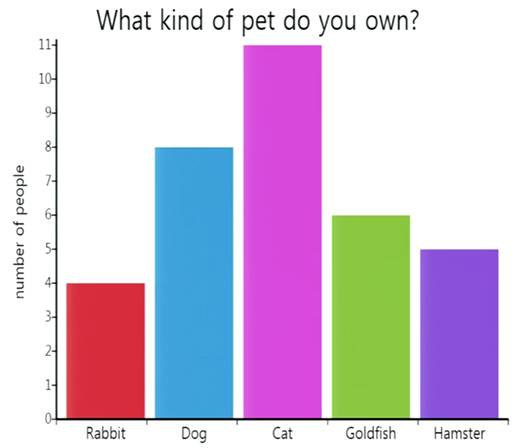# InfoCoBuild

## PSYC 60: Introduction to Statistics

PSYC 60: Introduction to Statistics (Winter 2016, UC San Diego). Instructor: Professor Adam J. Dede. This course provides an introduction to both descriptive and inferential statistics, core tools in the process of scientific discovery and the interpretation of research. Descriptive statistics are used to organize and describe sets of observations (data). Inferential statistics provide insight into characteristics of a population of interest based on a sample from that population. Emphasis will be on both conceptual understanding and calculation skills.Lecture 01 - Course Introduction, What is Statistics? Lecture 02 - Graphs and Average Lecture 03 - Measures of Variability, Characteristics of a Normal Distribution Lecture 04 - Correlation Lecture 05 - Regression Lecture 06 - Probability Lecture 07 - Sampling Distribution Lecture 08 - Review and Sampling Distribution Lecture 09 Lecture 10 - Hypothesis Testing Lecture 11 - T-test Lecture 12 - Power, Error, and Confidence Lecture 13 - T-tests: Two Independent Samples, Paired Samples, Repeated Samples Lecture 14 - T-tests: Two Independent Samples, Paired Samples, Repeated Samples Lecture 15 - Single Factor ANOVA Lecture 16 - Repeated Measures ANOVA Lecture 17 - ANOVA and Regression Lecture 18 - ANOVA and Regression Lecture 19 - Chi Squared Test Lecture 20 - Review

 Related Links PSYC 60 - Introduction to Statistics, Winter 2016 PSYC 60 - Introduction to Statistics - LE [B00] [VIDEO] - Prof. Dede, Adam Joseph - UCSD Winter 2016. Lecture 1 Media Files. Lecture 2 Media Files. ...